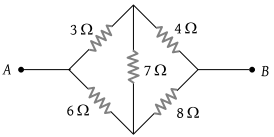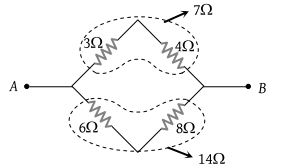# NEET Questions Solved

PMT - 2000

In the given figure, equivalent resistance between A and B will be(1) $\frac{14}{3}\text{\hspace{0.17em}\hspace{0.17em}}\Omega$

(2) $\frac{3}{14}\text{\hspace{0.17em}\hspace{0.17em}}\Omega$

(3) $\frac{9}{14}\text{\hspace{0.17em}\hspace{0.17em}}\Omega$

(4) $\frac{14}{9}\text{\hspace{0.17em}\hspace{0.17em}}\Omega$

(1) The given circuit is a balanced Wheatstone bridge, hence it can be redrawn as follows$⇒\text{\hspace{0.17em}\hspace{0.17em}\hspace{0.17em}\hspace{0.17em}\hspace{0.17em}\hspace{0.17em}}{R}_{eq}=\frac{7×14}{\left(7+14\right)}=\frac{14}{3}\Omega$.

Difficulty Level:

• 68%
• 13%
• 12%
• 10%